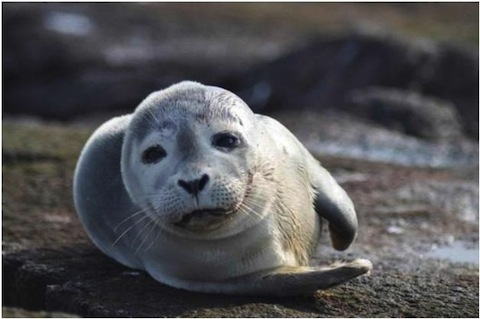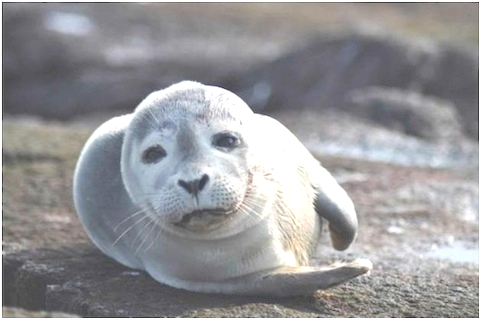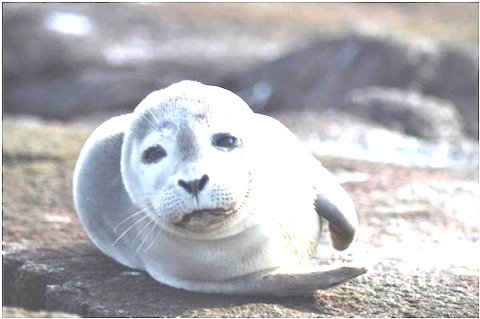### JavaScript Image Processing (3) - Brightness/Contrast Adjustment

24 Feb 2014

With the image class we created in previous posts, we are finally ready to have some fun with image processing. As a first step, let's try something simple.

Something simple huh? What can be simpler than linear transformation? I guess that's what we are targeting here: $$c_1 = \alpha \times c_0 + \beta$$ Simple as it is, we can still do something to make our images more appealing. Say we have somehow miscalculate the light and take a dark picture. A quick fix to it would be adjust the brightness of the image.Source Image Brightness Adjusted (+55) Image

Since the brightness of a pixel is proportional to its RGB values, it is obvious that we can change the brightness of a pixel by simply changing its RGB value. The brightness filter adjust the overall brightness level of an input image by adding to or subtracting from every pixel values directly. This corresponds to having $\alpha=1$ and $\beta\ne 0$ in linear transformation equation. To maintain the color consistency, the amount of change must be the same for every channel in every pixel.

var filters = {
'brightness' : function( src, val ) {
if( src.type === 'RGBAImage' ) {
var dc = new Color(val, val, val, 0);
return src.map(function( c ) {
return nc.clamp();
});
}
else {
throw "Not a RGBA image!";
}
}
};
The contrast filter shares a similar idea, while the difference is, in terms of the linear transformation equation, $\alpha\ne1$ and $\beta=0$. It is in fact a scaling of the pixel values. To set a boundary of the scaling, we take an input of contrast amount $C$ ranging from -128 to 128 and compute $\beta$ with this value $$\beta = \max\left(\frac{128+C}{128}, 0\right)$$
var filters = {
'contrast' : function( src, val ) {
if( src.type === 'RGBAImage' ) {
var factor = Math.max((128 + val) / 128, 0);
return src.map(function( c0 ) {
var c = c0.mulc(factor);
return c.clamp();
});
}
else {
throw "Not a RGBA image!";
}
}
};Source Image Contrast Adjusted (+55) Image

Putting them together, we arrive at the brightness/contrast filter:

var filters = {
'brightnesscontrast' : function( src, alpha, beta ) {
if( src.type === 'RGBAImage' ) {
var factor = Math.max((128 + val) / 128, 0);
var dc = new Color(beta, beta, beta, 0);
return src.map(function( c0 ) {
};Source Image Brightness (+55) and Contrast (+55) Adjusted Image